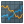You are viewing the RapidMiner Studio documentation for version 9.2 - Check here for latest version

#ARIMA (Time Series)

## Synopsis

This operator trains an ARIMA model for a selected time series attribute.

## Description

ARIMA stands for Autoregressive Integrated Moving Average. Typically an ARIMA model is used for forecasting time series.

An ARIMA model is defined by its three order parameters, p, d, q. p specifies the number of Autoregressive terms in the model. d specifies the number of differentations applied on the time series values. q specifies the number of Moving Average terms in the model.

An ARIMA model is an integrated ARMA model. The ARMA model describes a time series by a weighted sum of lagged time series values (the Autoregressive terms) and a weighted sum of lagged residuals. These residuals originates from a normal distributed noise process. The "integrated" indicates that the values of the ARMA model are integrated, which is equal to that the original time series values which the ARMA model describes are differentiated.

The ARIMA operator fits an ARIMA model with given p,d,q to a time series by finding the p+q coefficients (and if estimate constant is true, the constant) which maximize the conditional loglikelihood of the model describing the time series. For the optimization the LBFGS (Limited-memory Broyden-Fletcher-Foldfarb-Shanno) algorithm is used.

If chosing values for p,d,q, it is important that the conditional loglikelihood is only a good estimation for the exact loglikelihood if the number of parameters (sum of p,d,q) is not in the order of the length of the time series. Hence the number of parameters should be way smaller than the length of the time series.

How well a trained ARIMA model describes a given time series is often calculated with the Akaikes Information Criterion (AIC), the Bayesian Information Criterion (BIC) or a corrected Akaikes Information Criterion (AICC). The ArimaTrainer operator calculates these performance measures and outputs a Performance Vector containing the calculated values. An ARIMA model which describes a time series well has small information criteria.

This operator works only on numerical time series.

## Differentiation

This operator is similar to other modeling operators, but is specifically designed to work on time series data. One of the implications of this is, that the forecast model should be applied on the same data it was trained on.

###Apply Forecast

This operator receives a trained ARIMA model and create the forecast for the time series it was trained on.

## Input

•example set (IOObject)

The ExampleSet which contains the time series data as an attribute.

## Output

•forecast model (IOObject)

The ARIMA model (forecast model) fitted to the specified time series attribute. It also contains the original time series values.

•performance (Performance Vector)

This port delivers a performance vector of the fitted ARIMA model. The calculated performances are the AIC (Akaike information criterion), BIC ( Bayesian information criterion) and AICC (Akaike information criterion, corrected).

•original (IOObject)

The ExampleSet that was given as input is passed through without changes.

## Parameters

• time_series_attribute

The time series attribute (numerical) for which the ARIMA model should be build. The required attribute can be selected from this option. The attribute name can be selected from the drop down box of the parameter if the meta data is known.

Range:
• has_indices

This parameter indicates if there is an index attribute associated with the time series. If this parameter is set to true, the index attribute has to be selected.

Range:
• indices_attribute

If the parameter has indices is set to true, this parameter defines the associated index attribute. It can be either a date, date_time or numeric value type attribute. The attribute name can be selected from the drop down box of the parameter if the meta data is known.

Range:
• p:_order_of_the_autoregressive_model

The parameter p specifies the number of lags used by the autoregressive part of the ARIMA model.

Range:
• d:_degree_of_differencing

The parameter d specifies how often the time series values are differentiated.

Range:
• q:_order_of_the_moving-average_model

The parameter q specifies the order of the moving-average part of the model.

Range:
• estimate_constant

This parameter indicates if the constant of the ARIMA process should be estimated or not.

Range:
• main_criterion

The performance measure which is used as the main criterion in the Performance Vector.

• aic: Akaikes Information Criterion: Estimator of the relative quality of statistical models for a given set of data. The aic deals with the trade-off betwen the goodness of fit of the model and the simplicity of the model
• bic: Bayesian Information Criterion: Similar to the aic, but with a larger penalty term for the number of parameters in the model.
• aicc: corrected Akaikes Information Criterion: The aicc performance measure is the aic with a correction for small sample sizes, to prevent overfitting.
Range:

## Tutorial Processes

### Arima on Lake Huron Data

This tutorial process shows the basic usage of the ARIMA operator, by training an ARIMA model on the Lake Huron data set.### Arima on generated data

This tutorial process first generates data based on an ARIMA process. Then the ARIMA is applied to these data and creates a forecast model.### Auto Arima

In this tutorial process the Optimize Grid operator is used to find the best fitting ARIMA model to describe the Lake Huron data set.